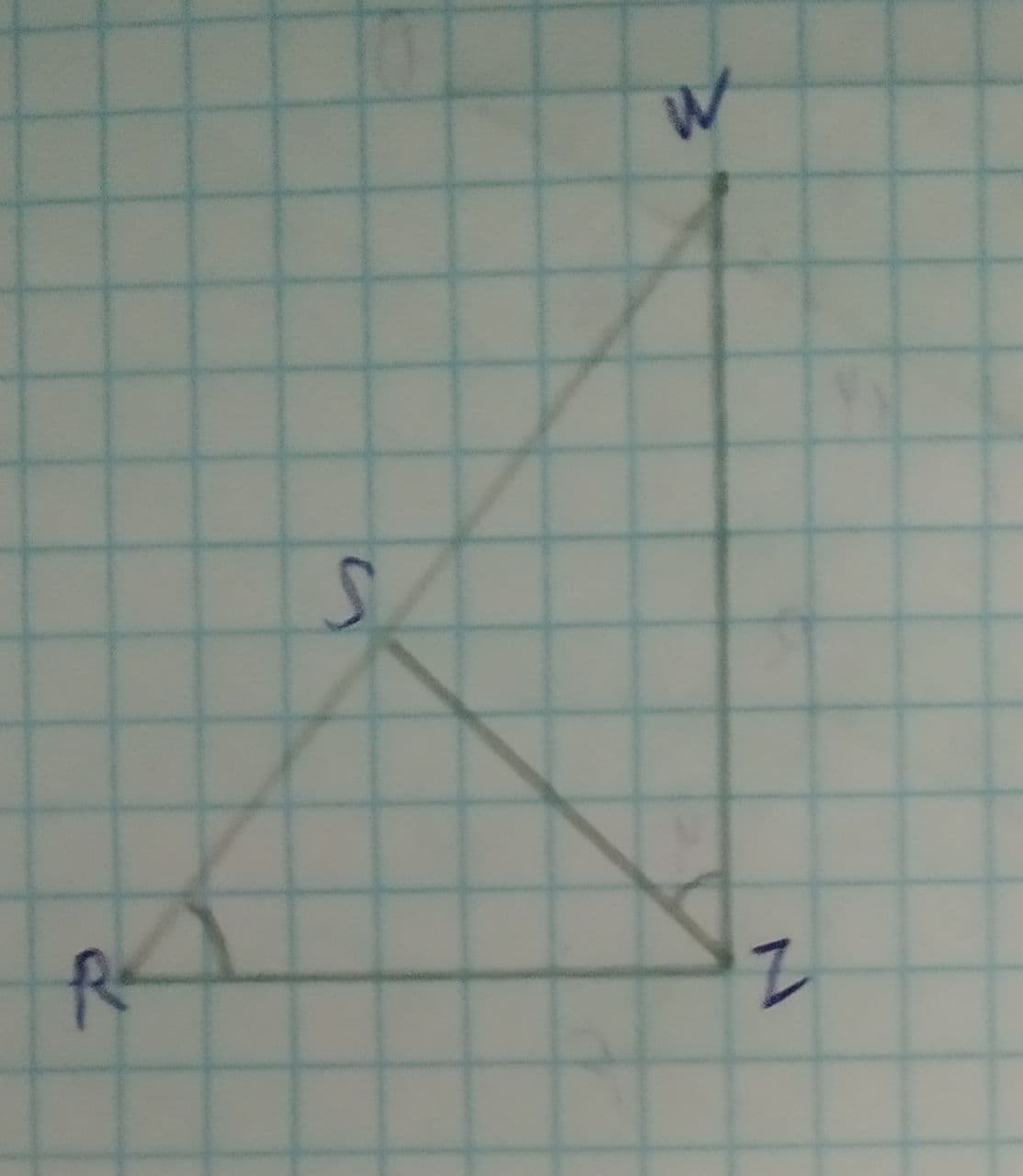# Complete statement \triangle RWZ = ? in the figure shown.ddaeeric 2021-08-09 Answered
To Complete: the statement $\mathrm{△}RWZ=$? in the figure shown.
Given:
Figure is shown below.You can still ask an expert for help

• Questions are typically answered in as fast as 30 minutes

Solve your problem for the price of one coffee

• Math expert for every subject
• Pay only if we can solve itwheezym
Calculation:
In .
$\mathrm{\angle }WRZ\stackrel{\sim }{=}\mathrm{\angle }WZS\therefore$ (Given)
$\mathrm{\angle }W\stackrel{\sim }{=}\mathrm{\angle }W\therefore$ (Common)
$WZ\stackrel{\sim }{=}WZ\therefore$ (Common)
By AAS similarity, $\mathrm{△}RWZ\sim \mathrm{△}ZWS$.
Therefore, the answer is $\mathrm{△}ZWS$.